NCERT Solutions for Class 10 Maths Chapter 5 Arithmetic Progressions Ex 5.1 are part of NCERT Solutions for Class 10 Maths. Here we have given NCERT Solutions for Class 10 Maths Chapter 5 Arithmetic Progressions Ex 5.1.

 Board CBSE Textbook NCERT Class Class 10 Subject Maths Chapter Chapter 5 Chapter Name Arithmetic Progressions Exercise Ex 5.1, Ex 5.2, Ex 5.3 Number of Questions Solved 4 Category NCERT Solutions

## NCERT Solutions for Class 10 Maths Chapter 5 Arithmetic Progressions Ex 5.1

Question 1.
In which of the following situations, does the list of numbers involved make an arithmetic progression and why?
(i) The taxi fare after each km when the fare is ₹ 15 for the first km and ₹ 8 for each additional km.
(ii) The amount of air present in a cylinder when a vacuum pump removes $$\frac { 1 }{ 4 }$$ of the air remaining in the cylinder at a time.
(iii) The cost of digging a well after every metre of digging, when it costs ₹ 150 for the first metre and rises by ₹ 50 for each subsequent metre.
(iv) The amount of money in the account every year, when ₹ 10000 is deposited at compound interest at 8% per annum.
Solution:
(i) Given:
a1 = ₹ 15
a2 = ₹ 15 + ₹ 8 = ₹ 23
a3 = ₹ 23 + ₹ 8 = ₹ 31
List of fares is ₹ 15, ₹ 23, ₹ 31
and a2 – a1 = ₹ 23 – ₹ 15 = ₹ 8
a3 – a2 = ₹ 31 – ₹ 23 = ₹ 8
Here, a2 – a1 = a3 – a2
Thus, the list of fares forms an AP.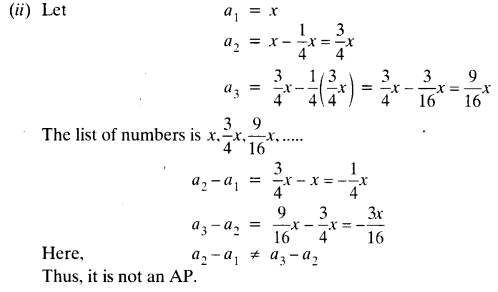(iii) Given:
a1 = ₹ 150, a2 = ₹ 200, a3 = ₹ 250
a2 – a1 = ₹ 200 – ₹ 150 = ₹ 50
and a3 – a2 = ₹ 250 – ₹ 200 = ₹ 50
Here, a3 – a2 = a2 – a1
Thus, the list forms an AP.
(iv) Given: a1 = ₹ 10000
a2 = ₹ 10000 + ₹ 10000 x $$\frac { 8 }{ 100 }$$ = ₹ 10000 + ₹ 800 = ₹ 10800
a3 = ₹ 10800 + ₹ 10800 x $$\frac { 8 }{ 100 }$$ = ₹ 10800 + ₹ 864 = ₹ 11664
a2 – a1 = ₹ 10800 – ₹ 10000 = ₹ 800
a3 – a2 = ₹ 11664 – ₹ 10800 = ₹ 864
a3 – a2 ≠ a2 – a1
Thus, it is not an AP.

Question 2.
Write first four terms of the AP, when the first term a and the common difference d are given as follows:
(i) a = 10, d = 10
(n) a = -2, d = 0
(iii) a = 4, d = -3
(iv) a = -1, d = $$\frac { 1 }{ 2 }$$
(v) a = -1.25, d = -0.25
Solution:
(i) Given: a = 10, d = 10
a1 = 10,
a2 = 10 + 10 = 20
a3 = 20 + 10 = 30
a4 = 30 + 10 = 40
Thus, the first four terms of the AP are 10, 20, 30, 40.

(ii) Given: a = – 2, d = 0
The first four terms of the AP are -2, -2, -2, -2.

(iii) a1 = 4, d = -3
a2 = a1 + d = 4 – 3 = 1
a3 = a2 + d = 1 – 3 = -2
a4 = a3 + d = -2 – 3 = -5
Thus, the first four terms of the AP are 4, 1, -2, -5.

(iv)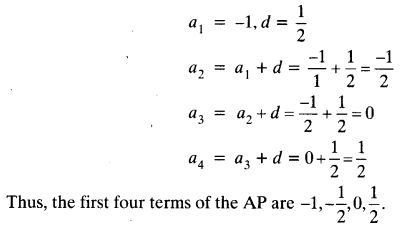(v) a1 = -1.25, d = -0.25
a2 = a1 + d = -1.25 – 0.25 = -1.50
a3 = a2 + d = -1.50 – 0.25 = -1.75
a4 = a3 + d = -1.75 – 0.25 = -2
Thus, the first four terms of the AP are -1.25, -1.50, -1.75, -2.

Question 3.
For the following APs, write the first term and the common difference:
(i) 3, 1, -1, -3, ……
(ii) -5, -1, 3, 7, ……
(iii) $$\frac { 1 }{ 3 }$$ , $$\frac { 5 }{ 3 }$$ , $$\frac { 9 }{ 3 }$$, $$\frac { 13 }{ 3 }$$ , ……..
(iv) 0.6, 1.7, 2.8, 3.9, …….
Solution:
(i) a1 = 3, a2 = 1
d = a2 – a1 = 1 – 3 = -2
where, a1 = first term and d = common difference
a1 = 3, d = -2
(ii) a1 = -5, a2 = -1
d = a2 – a1 = -1 – (-5) = -1 + 5 = 4
So, first term a1 = -5 and common difference d = 4
(iii) a1 = $$\frac { 1 }{ 3 }$$, a2 = $$\frac { 5 }{ 3 }$$
d = $$\frac { 5 }{ 3 }$$ – $$\frac { 1 }{ 3 }$$ = $$\frac { 4 }{ 3 }$$
So, first term a1 = $$\frac { 1 }{ 3 }$$ and common difference d = $$\frac { 4 }{ 3 }$$
a1= 0.6, a2 = 1.7
d = a2 – a1 = 1.7 – 0.6 = 1.1
So, first term a1 = 0.6 and common difference d = 1.1

Question 4.
Which of the following are APs ? If they form an AP, find the common difference d and write three more terms.
(i) 2, 4, 8, 16, …….
(ii) 2, $$\frac { 5 }{ 2 }$$ , 3, $$\frac { 7 }{ 2 }$$ , …….
(iii) -1.2, -3.2, -5.2, -7.2, ……
(iv) -10, -6, -2,2, …..
(v) 3, 3 + √2, 3 + 2√2, 3 + 3√2, …..
(vi) 0.2, 0.22, 0.222, 0.2222, ……
(vii) 0, -4, -8, -12, …..
(viii) $$\frac { -1 }{ 2 }$$ , $$\frac { -1 }{ 2 }$$ , $$\frac { -1 }{ 2 }$$ , $$\frac { -1 }{ 2 }$$ , …….
(ix) 1, 3, 9, 27, …….
(x) a, 2a, 3a, 4a, …….
(xi) a, a2, a3, a4, …….
(xii) √2, √8, √18, √32, …..
(xiii) √3, √6, √9, √12, …..
(xiv) 12, 32, 52, 72, ……
(xv) 12, 52, 72, 73, ……
Solution:
(i) 2, 4, 8, 16, ……
a2 – a1 = 4 – 2 = 2
a3 – a2 = 8 – 4 = 4
a2 – a1 ≠ a3 – a2
Thus, the given sequence is not an AP.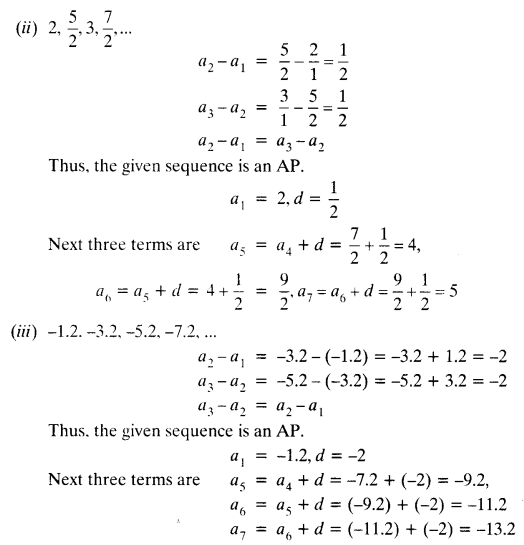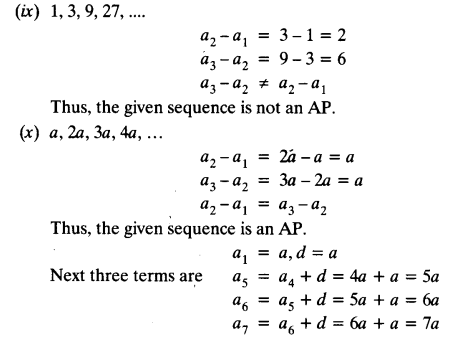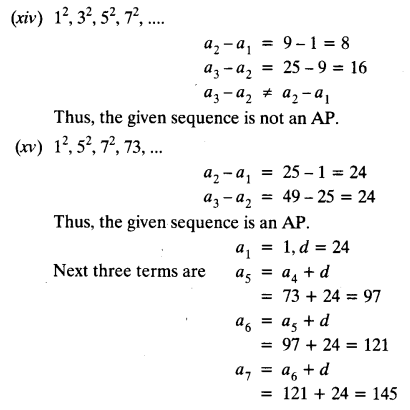We hope the NCERT Solutions for Class 10 Maths Chapter 5 Arithmetic Progressions Ex 5.1, help you. If you have any query regarding NCERT Solutions for Class 10 Maths Chapter 5 Arithmetic Progressions Ex 5.1, drop a comment below and we will get back to you at the earliest.